# Exponents: Help and Review Chapter Exam

Exam Instructions:

Choose your answers to the questions and click 'Next' to see the next set of questions. You can skip questions if you would like and come back to them later with the yellow "Go To First Skipped Question" button. When you have completed the practice exam, a green submit button will appear. Click it to see your results. Good luck!

### Page 1

#### Question 1 1. Which of the following would be true regarding the following exponential expression?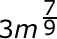#### Question 2 2. Which of the following is equivalent to the expression below?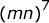#### Question 3 3. Which of the following is equivalent to the expression below?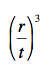#### Question 4 4. Which of the following is equivalent to the following expression?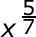#### Question 5 5. Which of the following is equivalent to the following expression?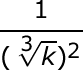### Page 2

#### Question 6 6. Simplify the expression below using positive exponents.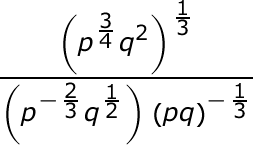#### Question 7 7. Simplify using only positive exponents.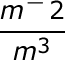#### Question 8 8. Which of the following is equivalent to the expression below?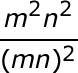#### Question 9 9. Which of the following radical expressions is equal to this exponential expression?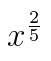#### Question 10 10. Simplify using ONLY positive exponents.### Page 3

#### Question 11 11. Simplify the following expression using positive exponents only.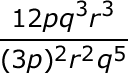#### Question 12 12. Simplify using ONLY positive exponents.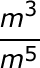#### Question 13 13. Simplify the following expression using positive exponents.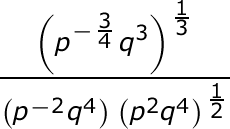#### Question 14 14. Which of the following exponential expressions is equal to this radical expression?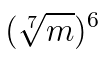#### Question 15 15. Simplify the following expression.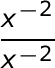### Page 4

#### Question 16 16. Simplify the following expression.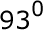#### Question 17 17. Using positive exponents, simplify the expression.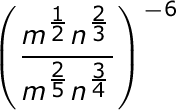#### Question 18 18. Which of the following is equivalent to the expression below?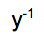#### Question 19 19. Simplify the expression below using positive exponents only.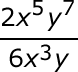#### Question 20 20. Simplify the expression using positive exponents.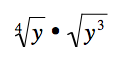### Page 5

#### Question 21 21. Simplify the expression below using only positive exponents.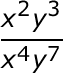#### Question 22 22. Simplify this expression using positive exponents.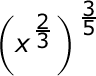#### Question 23 23. Simplify the following expression using positive exponents.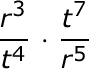#### Question 25 25. Simplify the expression below.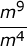#### Exponents: Help and Review Chapter Exam Instructions

Choose your answers to the questions and click 'Next' to see the next set of questions. You can skip questions if you would like and come back to them later with the yellow "Go To First Skipped Question" button. When you have completed the practice exam, a green submit button will appear. Click it to see your results. Good luck!

Support